# The BriefA Blog about the LSAT, Law School and Beyond

Sometimes, we want to multiply a number by itself several times. For example, if we are trying to guess someone’s password, we may want to know how many 5-letter sequences are possible. Following our lessons on combinatorics (see here), we know that there arepossibilities. But we may want an easier way to write this down. The solution is to use exponents. In general,

Rule for Positive Exponents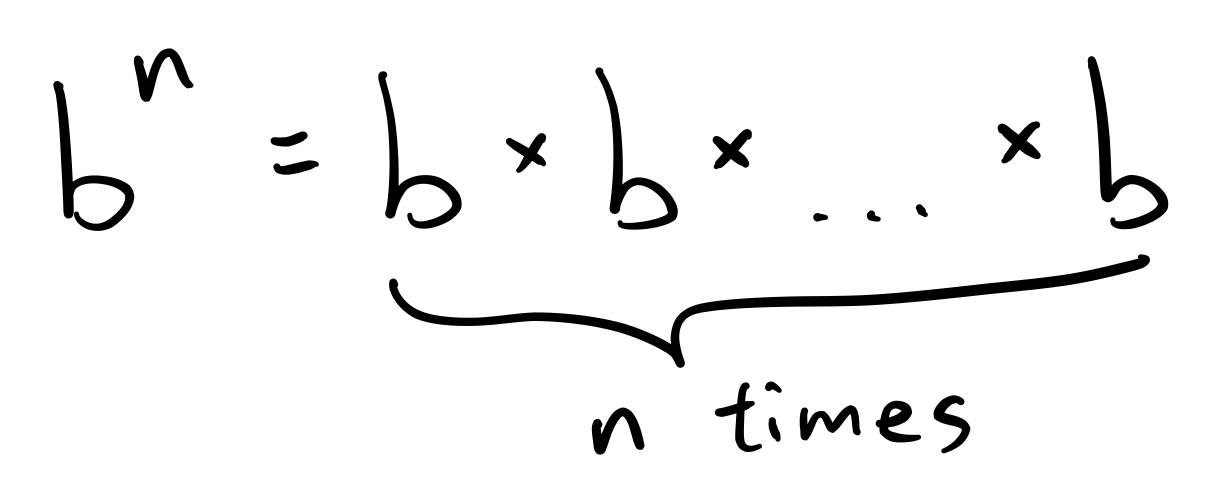Using this rule, we can rewrite our earlier expression as simplyWe call 26 the base and we call 5 the exponent or power. And in general, for,is the base andis the exponent. In English, we say this is “b to the n-th power.” So we would say thatis 26 to the 5th power.

Now, we can take any number and raise it to the power of any positive integer simply by multiplying the number by itself that many times. So, we have: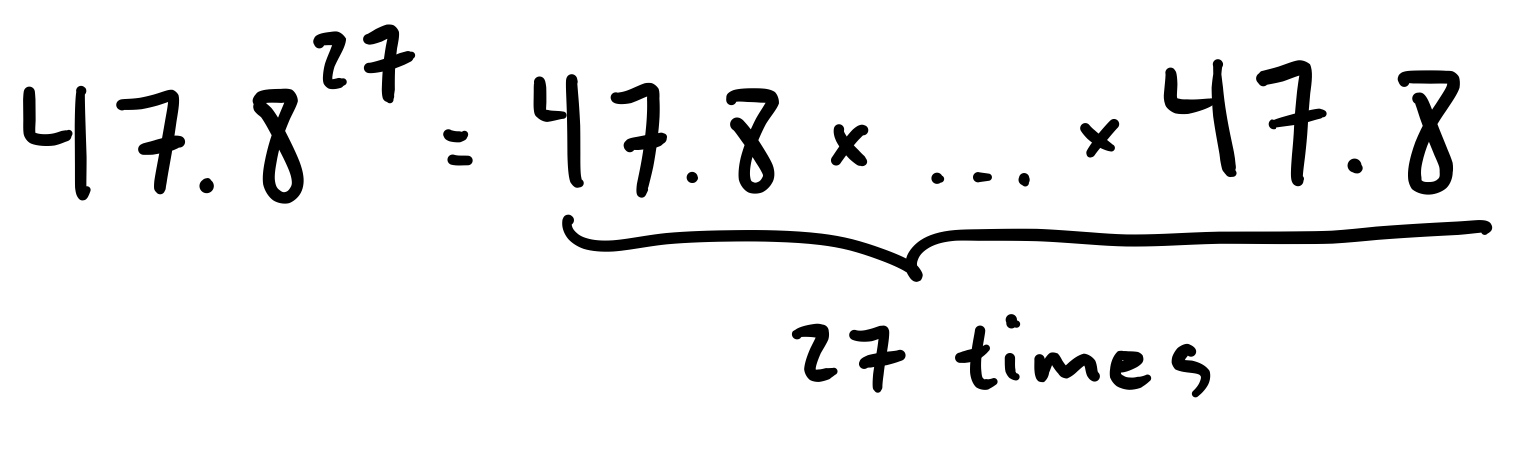We can even do this for irrational numbers, like: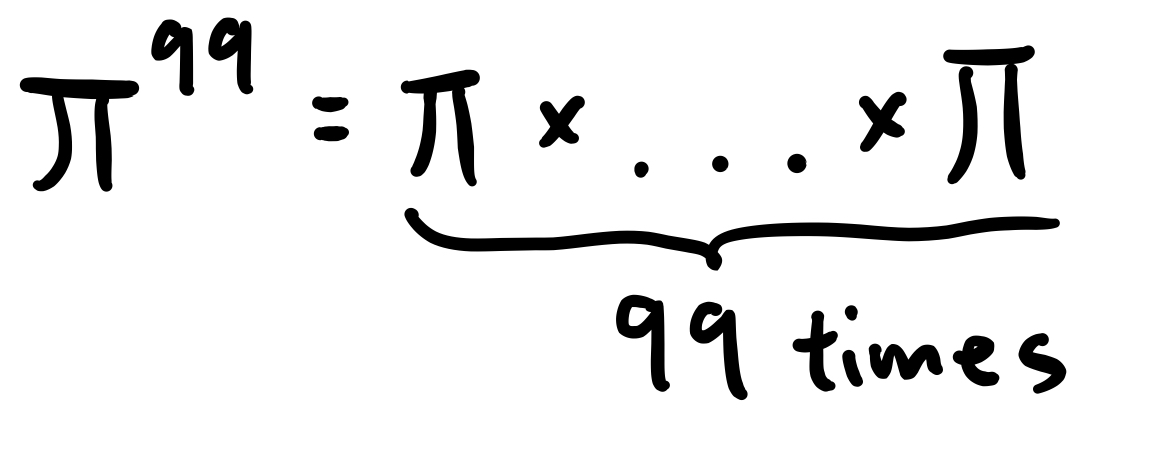But we can also have negative exponents. When we take a negative exponent, we use the following formula:

Rule for Negative ExponentsIn essence, we flip the base and then take a normal exponent. Here are some examples:Finally, if we raise anything (other than 0) to the 0th power, we get 1. In other words,

Rule for the 0th Power

IfthenFor example:Now, there are some rules for how different things with exponents can be combined.

Multiplication and Division Rules for ExponentsExponent Rule for ExponentsPractice Problems

Simplify the following expressions:

1.2.3.4.5.6.Comment on this

Linear equations are a specific kind of equation that meets certain limitations. Specifically, linear equations are those where every variable (if there are any variables at all) is raised only to the first power (see here for a guide to powers/exponents). Furthermore, those variables must not be multiplied by any other variables.

Here are some examples of linear equations:Here are some examples of equations that are not linear:In subsequent posts, we will discuss two methods for solving linear equations: elimination and substitution. We will also talk about systems of linear inequalities, which occur when we replace those equality signs with inequalities likeor.

For now, see if you can identify whether the following equations are linear or not:

Practice Problems:

Identify whether the following equations are linear or not

1.2.3.4.5.6.Now, systems of linear equations are just groups of 1 or more linear equations. The solutions to the system are all the different ways of assigning values to each variable such that every equation in the group is true. Note that every solution must specify the value for each variable in our system. So, for example, in the following system:The responseis not a solution. However,is a solution. And in general, we can give our solutions in the form of ordered pairs. So we would write (2, 3) is a solution, where the first coordinate corresponds to the value ofand the second coordinate corresponds to the value of.

The reason for requiring that solutions specify values for all variables (not just some) is that sometimes a certain value for one variable can be a solution and sometimes not, depending on the values of the other variables. Consider:Now, this system has many solutions. For example,is a solution. But we cannot simply say thatis a solution, for if, then that is no solution at all (since)! Thus, we require that a solution for a system of equations specify values for every variable in the system.

In our next post, we discuss how to solve systems of linear equations via elimination.

Comment on this

In this series, we discuss how to solve systems of equations. This is an important skill for the GRE since many of the math problems will involve solving systems of equations (e.g. problems with inequalities, algebra problems, or word problems). Now, this content builds on some other mathematical ideas, like the idea of a variable and the distributive law. If you want a refresher on those ideas, see this post.

What is a “system of equations”?

A system of equations is a group of one or more equations, like this:Or this:And we want to find the possible value(s) of x and any other variables in the problem. So, for example, in the second pair of equations we could have:And then we would get:making both equations true. But if we hadthen we would get:which would make the second equation true, but not the first. A solution to a system of equations is an assignment of values to every variable such that every equation in the system is true. By what we have said above,is a solution to the above pair of equations, whereasis not.

In this series, we will focus on solving two basic kinds of problems:

1. Linear Systems of Equations: These only have variables raised to the 1st power. For example:
••2. Quadratic Systems of Equations: These include variables raised to the 2nd power. For example:
••What Kinds of Problems Involve This Skill?

This skill can come in handy for many different types of GRE problems, such as:

• Quantity Comparison Problems
Suppose. Which of the following is larger?• Word Problems
Tom and Liz are baking cookies. Tom can bake 10 cookies in an hour and Liz can bake 5 cookies in an hour. Working together, how long will it take them to bake 40 cookies?
• Problems with Infinite Series
Suppose we have the series: 1, ½, ¼, …
What is its sum?
• Problems with Inequalities
Which values ofwill satisfy the following system of inequalities?In our next post, we discuss what a linear equation is, a precursor to actually solving them

Comment on this

In order to solve systems of equations, we need to understand three concepts: variables, how to manipulate equations, and the distributive law.

Variables

Variables are letters that stand in for numbers. Often, we will use the lettersas variables. We can use equations to assign a specific value to a certain variable. For example,means that the variableis equal to the number.

We use variables to capture certain relationships between quantities when we do not know the specific values involved. For example, I could tell you that my cell phone is worth twice as much as my laptop (and this is true). Without variables, we would have no way to represent this mathematically, since we do not know the actual value of either my laptop or cell phone. But with variables, we can say:the value of my laptop,the value of my cell phone. Then, we can represent this information mathematically as:Thus, by using variables, we can capture relationships between unknown values.

Manipulating Equations

The second idea we need to understand is how to manipulate equations. Often, we are given a complicated equation like:And we want to turn it into a simpler equation, likeNow, the key is that we are allowed to anything we like to one side of the equation provided we do it to the other side as well. So, for example, we can take our initial equationAnd we can subtract 8 from both sides to get:And we can divide by 3, provided we do it to both sides, to get:So now we know what the value ofis, namely 3.

The reason why this works is that in an equation, you are saying that the left hand side is equal to the right hand side. In other words, that the left hand side represents the very same number as the right hand side. So in our equation,We are saying thatis just another way of writing the number 17. So of course we can do anything we like to both sides and the resulting numbers will still be equal, since we are doing the same thing to the same numbers.

Remember, though, that we still cannot do mathematically illicit operations, like dividing by 0, even if we do them to both sides.

Distributive Law

Finally, we need to understand how the distributive law works. It is a rule for how multiplication and addition interact and it states:An example may help in seeing that the distributive law is, in fact, true:

We know that, and we know thatbecause operations in parenthesis are done before any other operations. Thus, we know thatAnd by the distributive law,So it is true thatsinceand(and of course).

Now, one very important application of the distributive law is used in factoring quadratic equations (as we discuss here). We will need to apply to the distributive law to:By distributing, we get:And in general, we will omit the multiplication symboland simply write:You may have also seen this formula called FOIL (First Outer Inner Last), a mnemonic meant to remind people of the four terms in our equation: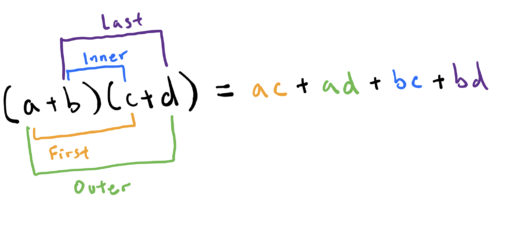However you choose to remember it, this formula is an important part of understanding how to factor equations.

Comment on this

The T-14.

It seems like no one talks about anything else. But where did this term come from and what does it actually measure?

### Where the Term "T-14" Came From

The term "T-14" refers to the top fourteen law schools according to U.S. News and World Report (USNWR). But why fourteen instead of ten or twenty-three or five? Because the top fourteen schools have stayed fairly consistent according to USNWR.

Let's repeat that for emphasis: they've stayed consistent according to USNWR.

But USNWR's rankings are somewhat arbitrary. Here's how USNWR determines them: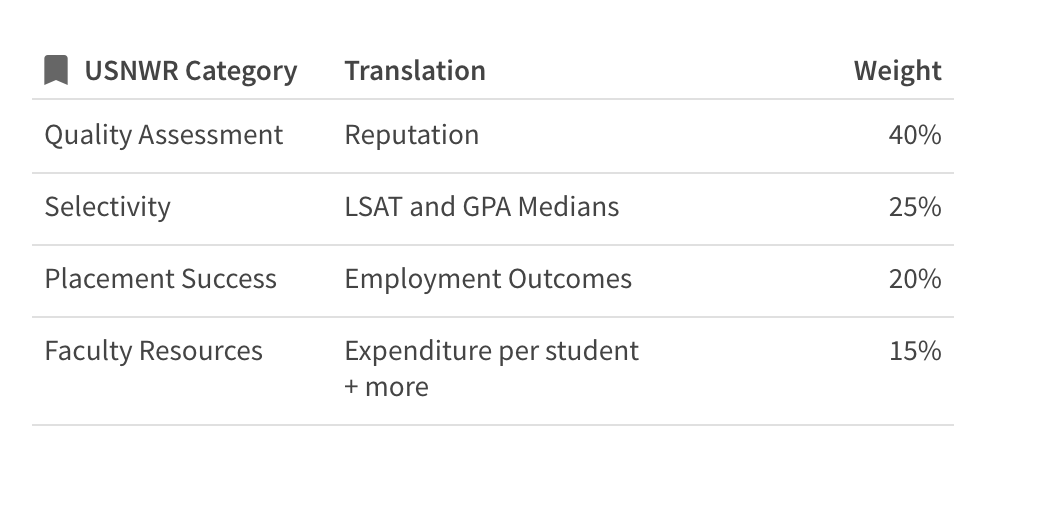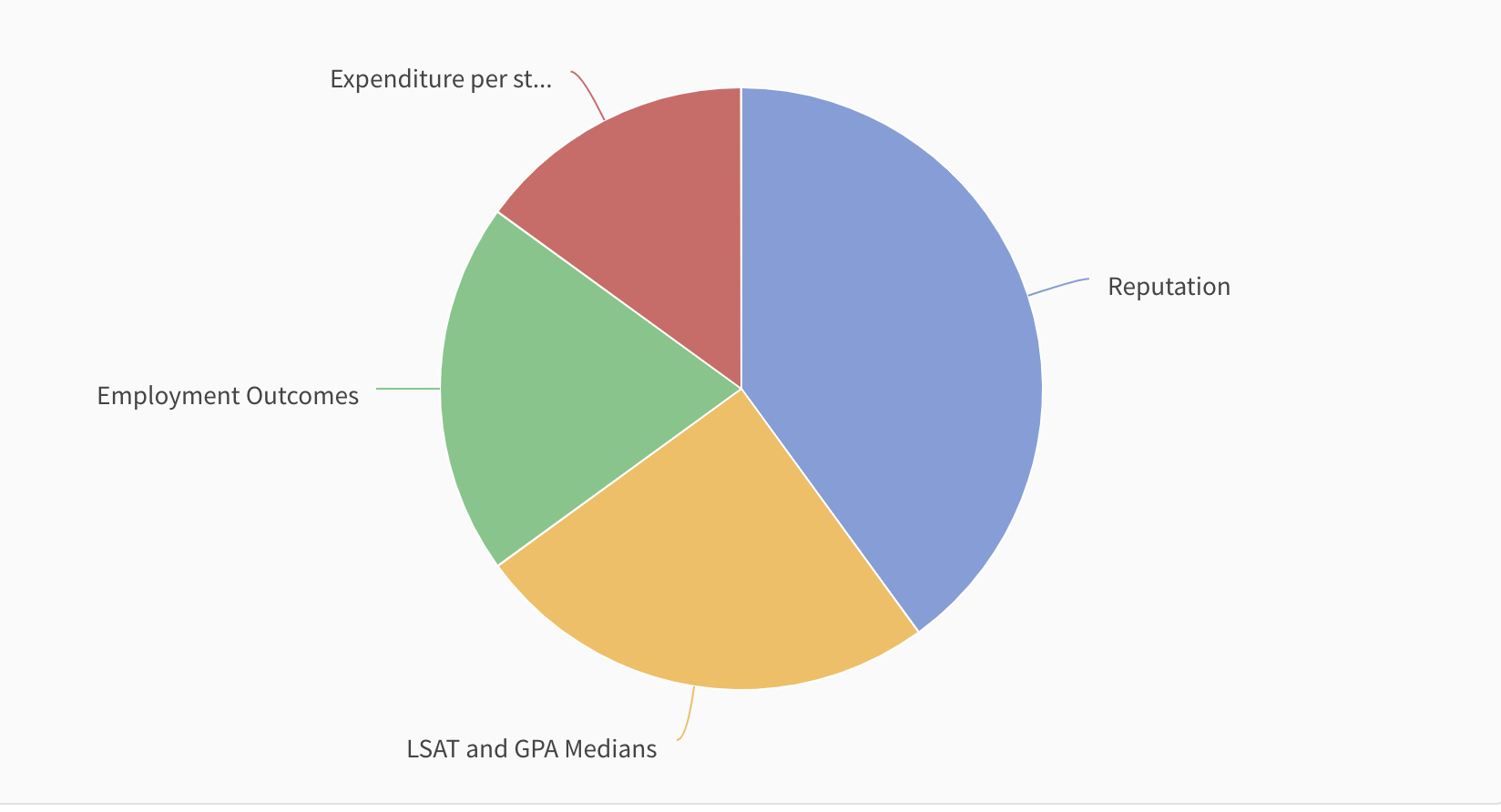Notice that USNWR rankings are mostly a measure of reputation. This is a little odd, because USNWR also helps perpetuate these schools' reputations.

To some extent, the rankings are a self-fulfilling prophecy: the longer you maintain a high USNWR rank, the better your reputation…and the higher your USNWR rank. No wonder they stay static at the top.

It's also worth pointing out what the rankings are not. They are not primarily a measure of employment outcomes. They are not at all a measure of how prepared you'll be to succeed. "Faculty resources" is a poor stand-in for educational quality, as you can see in a more detailed breakdown of the ranking methodology: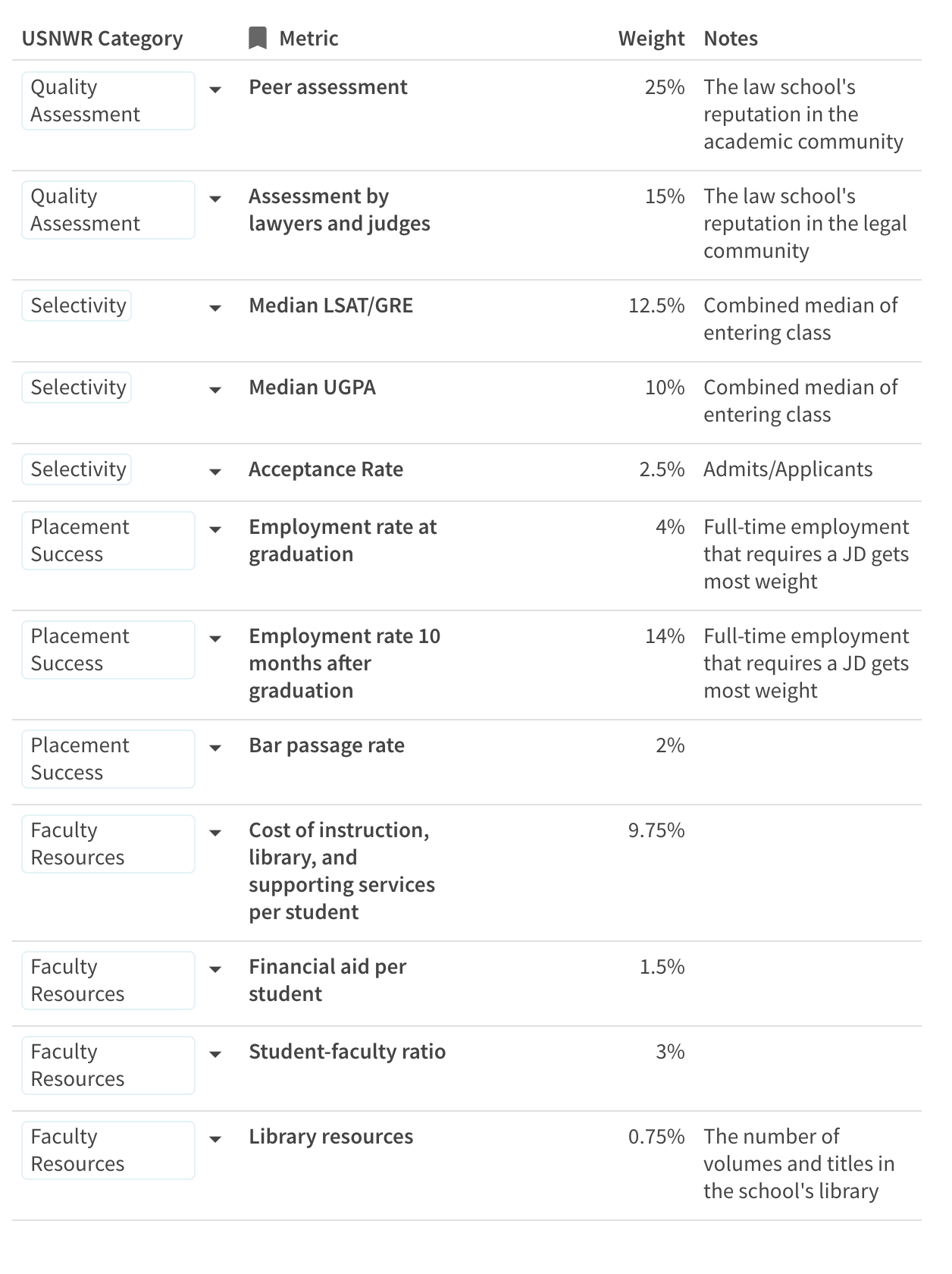Let's question some of these assumptions. Does the fact that a school spends more on its instructors, library, and supporting services mean that it is better? Maybe—or maybe it's just in a more expensive location. Does the amount a school spends on its instructors and library and supporting services really matter more than twice as much as the students' employment rate at graduation? Do you, or does anyone you know, care even 0.75% about how many volumes are in the school's library?

The point isn't that these rankings are bad. The point is that rankings—any rankings—necessarily rely on assumptions that may not hold true for you.

So, back to the most important question:

### Does attending a T-14 school matter?

It depends on what you're looking for.

Graduating from a highly ranked school usually makes it easier to do the following:

• Get a job in Big Law—especially the first time around
• Get a federal clerkship

But graduating from a T-14 school does not guarantee a job, and graduating from a school of lower ranking doesn't mean you can't get a job in Big Law. Your class rank, network, and interviewing skills also matter a lot.

If you're contemplating a specific school, you should take a look at the latest ABA-disclosed employment outcomes.

Comment on this

We’ve rounded up five spectacular personal statements that helped students with borderline numbers get into T-14 schools. You’ll find these examples to be as various as a typical JD class. Some essays are about a challenge, some about the evolution of the author’s intellectual or professional journey, and some about the author’s identity. The only common thread is sincerity. The authors did not write toward an imagined idea of what an admissions officer might be looking for: they reckoned honestly with formative experiences.

Featured image: Person Holding Blue Ballpoint Pen Writing in Notebook by picjumbo.com via Pexels is free to use without attribution.

At 7Sage, we have worked with hundreds of law school applicants from China, South Korea, and many more countries, and we have assembled the following FAQ to help international students gain admission to America’s top law schools.

Featured image: Close-up of World Map by Pixabay via Pexels is free to use without attribution.

1 comment

An LL.M. is a one-year master’s degree for candidates who already have a degree in law, and it can help them switch to a new field of law, get a new job, or gain a professional edge. Read about why you might apply and how to maximize your chances in our admissions course: https://7sage.com/admissions/lesson/all-about-ll-m-degrees/.

Comment on this

On today's episode David Busis, co-founder of 7Sage Admissions Consulting, speaks with Elizabeth Cavallari, an ex-admissions dean of admissions at William & Mary Law School about how to manage the waitlist.

Comment on this

The annual uproar about law school rankings might lead you to believe that the rank of the school you attend is the only factor in determining whether you will become a successful lawyer. As Above The Law points out, the T14 law school rankings, as determined by US News and World Report, rely heavily on inputs – especially peer assessment, grades, and LSAT scores — while ATL’s rankings rely more heavily on outputs like jobs and starting salaries. Given that the two lists overlap quite heavily at the top, I wouldn’t blame you for feeling like you might as well say goodbye to your law career before you’ve even read your first case note if you don’t get into a T14 school. But don’t lose heart! Many, many law school graduates attend non-T14 schools and go on to have successful law careers.

I speak from experience. By way of background, I graduated from Emory Law School squarely in the middle of my class. It was a great place to go to school, with whip-smart professors and clinics, but it was not T14 when I attended and still isn’t (though it’s been solidly T25 for many years). Emory is also located in Atlanta, which, for all of its charms, was not the city where I intended to practice upon graduation. Like so many others, I had my eyes set on New York City. I managed to write myself onto the law review which, given my highly mediocre class ranking, definitely helped boost my resumé. This, combined with my comfort with interviewing, helped me land a job in Big Law in the New York office of a Chicago-based firm, where I specialized in real estate law.

I jumped ship after five years and wound up in Cardozo’s admissions office, where I counseled prospective students about whether they should or shouldn’t go to law school, and why they might be a good fit for Cardozo in particular. I later returned to practicing real estate law with the New York City Economic Development Corporation. As a lawyer, first in private practice and later for the City of New York, I regularly interviewed candidates for summer associate and lateral positions. While I can’t speak for every law firm or government agency, I do think I have some insight about whether attending a T14 law school really matters—so here goes!